# New to Qlik Sense

If you’re new to Qlik Sense, start with this Discussion Board and get up-to-speed quickly.

Announcements
Join “Do More With Qlik” with Mike Tarallo to get a first-hand look at the New Authoring Experience, June 29th: REGISTER HERE
cancel
Showing results for
Did you mean: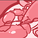Contributor

## How to create a chart with multiple aggregation grouping?

This is my first app with Qlik. I am trying to create a chart where I can see the Hour of the day where I had the max Count. To calculate the max Count first I have to aggregate the Sum of count for each hour.

What kind of chart can be used to achieve this? Or How can I manipulate the data before using it in the chart?

My data looks like this. Here for example rows 2 to 6 contains the count for hour 8. I need to sum by hour and find the max for each date.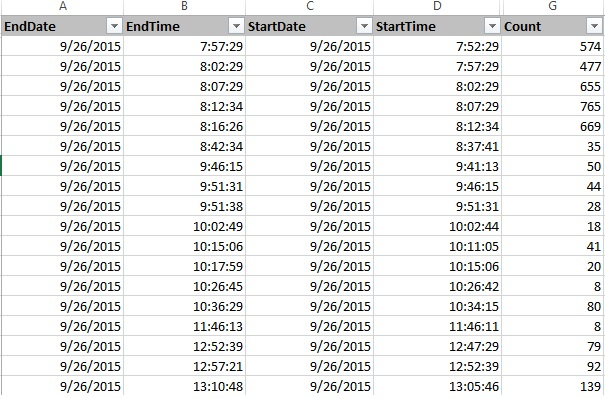I would like to see an output something like this. Where the dot represent the hour of the day that has max count.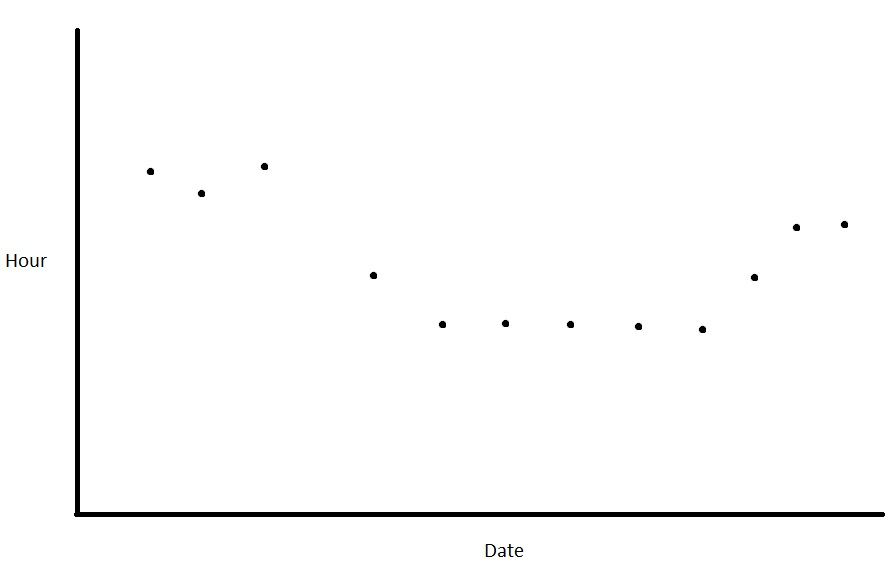1 Solution

Accepted SolutionsSpecialist II

I don't see in Sense graphics with point instead of line,

so this one should wotk :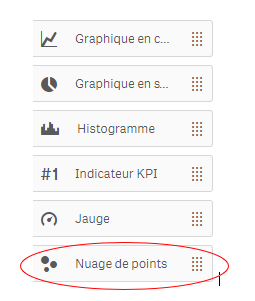as dimension :

StartDate

as Measures :

X-axis : only(StartDate)

Y-axis : expression above

regards

6 RepliesSpecialist II

Hi,

regardsContributor
AuthorSpecialist II

Hi,

first in script, add a field StartH as Hour(StartTime)

then in front :

graph with StartDate as dimension

and expression :

FirstSortedValue(total <StartDate> StartH,aggr(sum(Count),StartDate,StartH))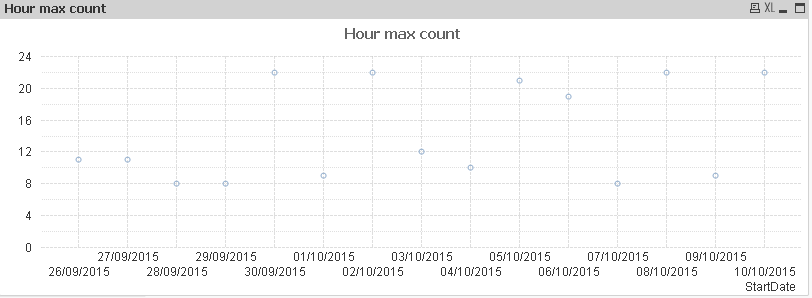thank's for the sample !Specialist II

correction !!

I allways forget : firstsortedvalue gives the min, must change sign to have the max :

FirstSortedValue(total <StartDate> StartH,-aggr(sum(Count),StartDate,StartH))

(minus sign before aggr(sum

so it's better :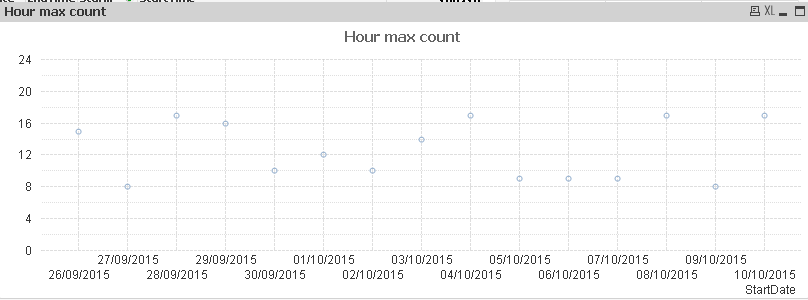Contributor
Author

Thanks Olivier.

So both my X and Y axis will be a Dimension right? Which Chart Type should by using here?Specialist II

I don't see in Sense graphics with point instead of line,

so this one should wotk :as dimension :

StartDate

as Measures :

X-axis : only(StartDate)

Y-axis : expression above

regards